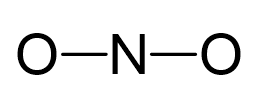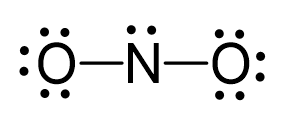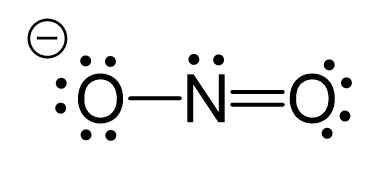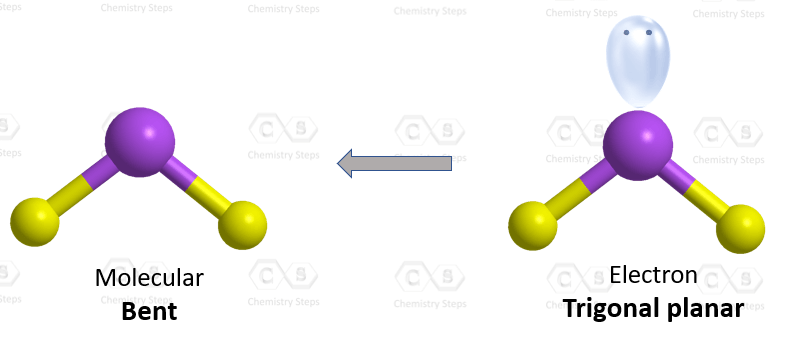## Examples

Nitrogen is the central atom:There are 5 + 2×6 + 1 = 18 electrons, and 4 are used to make the two covalent bonds. Both oxygens get 6 electrons as three lone pairs, and nitrogen gets one lone pair:One lone pair from an oxygen is used to make a π bond with the nitrogen and thus making the ionic charge -1:The central atom has two atoms and a lone pair; therefore, the electron geometry is trigonal planar, and the molecular geometry is bent.The steric number (the sum of the number of the atoms and lone pairs) of the carbon is 3 which corresponds to sp2-hybridization.

More practice examples on geometry and hybridization in this multiple-choice quiz:

Check Also